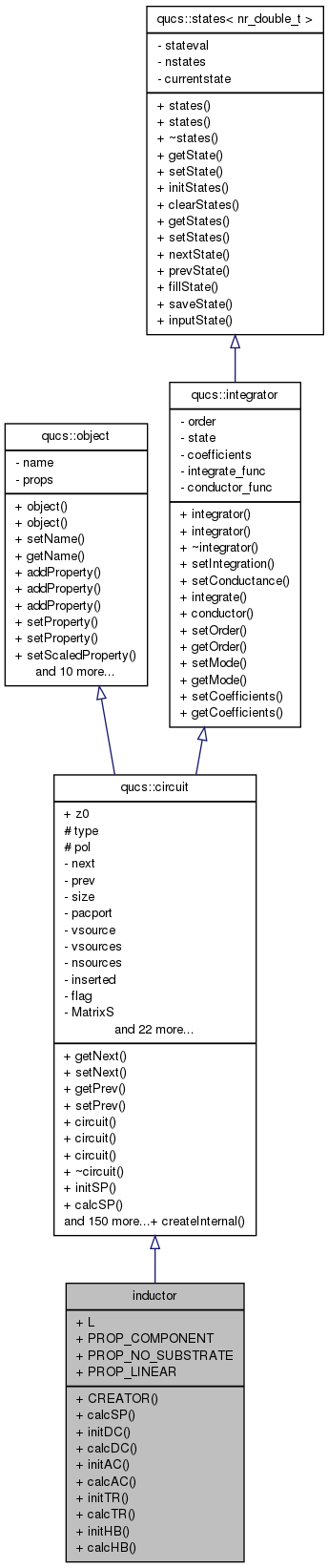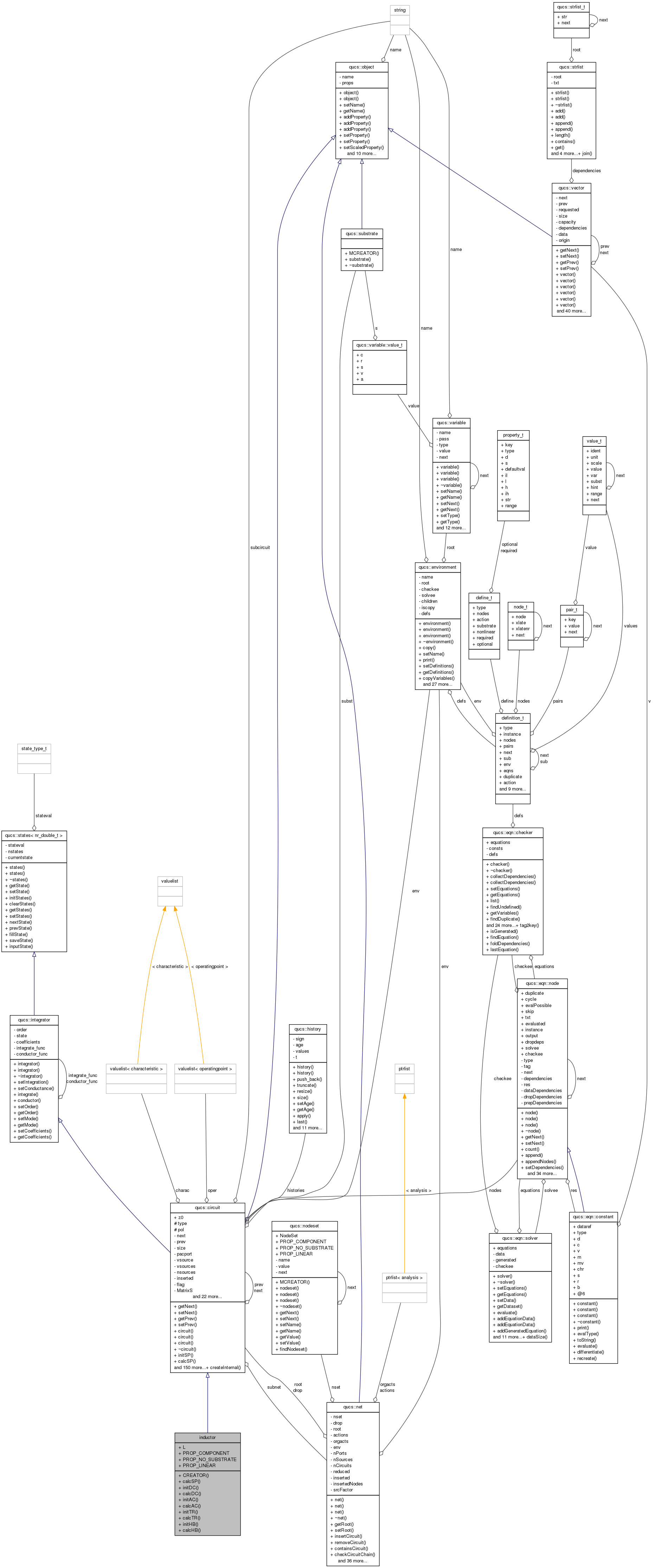Qucs-core  0.0.19
inductor Class Reference

`#include <inductor.h>`

Inheritance diagram for inductor:[legend]
Collaboration diagram for inductor:[legend]

## Public Member Functions

CREATOR (inductor)
void calcSP (nr_double_t)
void initDC (void)
void calcDC (void)
void initAC (void)
void calcAC (nr_double_t)
void initTR (void)
void calcTR (nr_double_t)
void initHB (void)
void calcHB (nr_double_t)

## Data Fields

L
PROP_COMPONENT
PROP_NO_SUBSTRATE
PROP_LINEAR

## Detailed Description

Definition at line 126 of file inductor.cpp.

## Member Function Documentation

 void inductor::calcAC ( nr_double_t frequency ) ` [virtual]`

Reimplemented from qucs::circuit.

Definition at line 73 of file inductor.cpp.

 void inductor::calcDC ( void ) ` [virtual]`

Reimplemented from qucs::circuit.

Definition at line 54 of file inductor.cpp.

 void inductor::calcHB ( nr_double_t frequency ) ` [virtual]`

Reimplemented from qucs::circuit.

Definition at line 116 of file inductor.cpp.

 void inductor::calcSP ( nr_double_t frequency ) ` [virtual]`

Reimplemented from qucs::circuit.

Definition at line 39 of file inductor.cpp.

 void inductor::calcTR ( nr_double_t ) ` [virtual]`

Reimplemented from qucs::circuit.

Definition at line 93 of file inductor.cpp.

 inductor::CREATOR ( inductor )
 void inductor::initAC ( void ) ` [virtual]`

Reimplemented from qucs::circuit.

Definition at line 58 of file inductor.cpp.

 void inductor::initDC ( void ) ` [virtual]`

Reimplemented from qucs::circuit.

Definition at line 48 of file inductor.cpp.

 void inductor::initHB ( void ) ` [virtual]`

Reimplemented from qucs::circuit.

Definition at line 109 of file inductor.cpp.

 void inductor::initTR ( void ) ` [virtual]`

Reimplemented from qucs::circuit.

Definition at line 84 of file inductor.cpp.

## Field Documentation

Definition at line 127 of file inductor.cpp.

Definition at line 127 of file inductor.cpp.

Definition at line 127 of file inductor.cpp.

Definition at line 127 of file inductor.cpp.

The documentation for this class was generated from the following files: